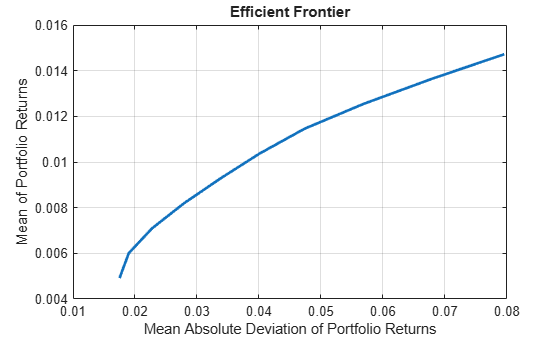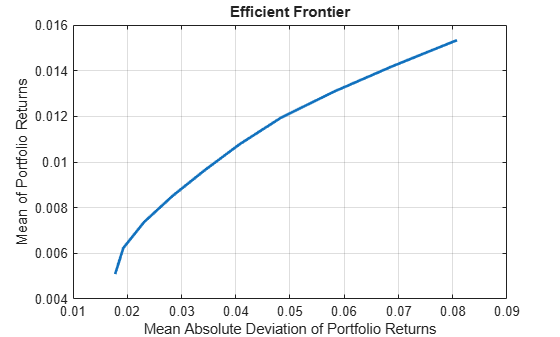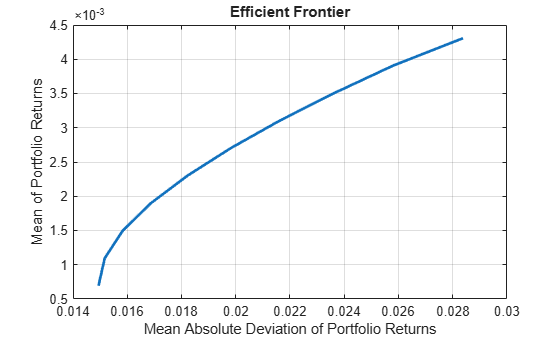Create PortfolioMAD object for mean-absolute deviation portfolio optimization and analysis

## Description

The `PortfolioMAD` object implements mean-absolute deviation portfolio optimization, where MAD stands for “mean-absolute deviation.” `PortfolioMAD` objects support functions that are specific to MAD portfolio optimization.

The main workflow for MAD portfolio optimization is to create an instance of a `PortfolioMAD` object that completely specifies a portfolio optimization problem and to operate on the `PortfolioMAD` object to obtain and analyze efficient portfolios. For more information on the workflow when using `PortfolioMAD` objects, see PortfolioMAD Object Workflow.

You can use the `PortfolioMAD` object in several ways. To set up a portfolio optimization problem in a `PortfolioMAD` object, the simplest syntax is:

`p = PortfolioMAD;`
This syntax creates a `PortfolioMAD` object, `p`, such that all object properties are empty.

The `PortfolioMAD` object also accepts collections of name-value pair arguments for properties and their values. The `PortfolioMAD` object accepts inputs for properties with the general syntax:

` p = PortfolioMAD('property1',value1,'property2',value2, ... );`

If a `PortfolioMAD` object exists, the syntax permits the first (and only the first argument) of the `PortfolioMAD` object to be an existing object with subsequent name-value pair arguments for properties to be added or modified. For example, given an existing `PortfolioMAD` object in `p`, the general syntax is:

`p = PortfolioMAD(p,'property1',value1,'property2',value2, ... );`

Input argument names are not case-sensitive, but must be completely specified. In addition, several properties can be specified with alternative argument names (see Shortcuts for Property Names). The `PortfolioMAD` object tries to detect problem dimensions from the inputs and, once set, subsequent inputs can undergo various scalar or matrix expansion operations that simplify the overall process to formulate a problem. In addition, a `PortfolioMAD` object is a value object so that, given portfolio `p`, the following code creates two objects, `p` and `q`, that are distinct:

`q = PortfolioMAD(p, ...)`

After creating a `PortfolioMAD` object, you can use the associated object functions to set portfolio constraints, analyze the efficient frontier, and validate the portfolio model.

For more detailed information on the theoretical basis for conditional value-at-risk portfolio optimization, see Portfolio Optimization Theory.

## Creation

### Syntax

``p = PortfolioMAD``
``p = PortfolioMAD(Name,Value)``
``p = PortfolioMAD(p,Name,Value)``

### Description

example

````p = PortfolioMAD` creates an empty `PortfolioMAD` object for mean-absolute deviation portfolio optimization and analysis. You can then add elements to the `PortfolioMAD` object using the supported "add" and "set" functions. For more information, see Creating the PortfolioMAD Object.```

example

````p = PortfolioMAD(Name,Value)` creates a `PortfolioMAD` object (`p`) and sets Properties using name-value pairs. For example, ```p = PortfolioMAD('AssetList',Assets(1:12))```. You can specify multiple name-value pairs.```

example

````p = PortfolioMAD(p,Name,Value)` creates a `PortfolioMAD` object (`p`) using a previously created `PortfolioMAD` object `p` and sets Properties using name-value pairs. You can specify multiple name-value pairs.```

### Input Arguments

expand all

Previously constructed `PortfolioMAD` object, specified using `PortfolioMAD`.

expand all

## Setting Up the Object

Names or symbols of assets in the universe, specified as a cell array of character vectors or a string array.

Data Types: `cell` | `string`

Initial portfolio, specified as a vector.

Data Types: `double`

Name for instance of the `PortfolioMAD` object, specified as a character vector.

Data Types: `char` | `string`

Number of assets in the universe, specified as an integer scalar.

Data Types: `double`

## Portfolio Object Constraints

Linear equality constraint matrix, specified as a matrix.

Data Types: `double`

Linear inequality constraint matrix, specified as a matrix.

Data Types: `double`

Linear equality constraint vector, specified as a vector.

Data Types: `double`

Linear inequality constraint vector, specified as a vector.

Data Types: `double`

Group A weights to be bounded by weights in group B, specified as a matrix.

Data Types: `double`

Group B weights, specified as a matrix.

Data Types: `double`

Group membership matrix, specified as a matrix.

Data Types: `double`

Lower-bound constraint, specified as a vector.

Data Types: `double`

Lower-bound budget constraint, specified as a scalar.

Data Types: `double`

Lower-bound group constraint, specified as a vector.

Data Types: `double`

Minimum ratio of allocations between `GroupA` and `GroupB`, specified as a vector.

Data Types: `double`

Upper-bound constraint, specified as a vector.

Data Types: `double`

Upper-bound budget constraint, specified as a scalar.

Data Types: `double`

Upper-bound group constraint, specified as a vector.

Data Types: `double`

Maximum ratio of allocations between `GroupA` and `GroupB`, specified as a vector.

Data Types: `double`

Type of bounds for each asset weight, specified as a scalar character vector or string, or a cell array of character vectors or a string array. For more information, see `setBounds`.

Data Types: `char` | `cell` | `string`

Minimum number of assets allocated in portfolio, specified as a scalar numeric value. For more information, see `setMinMaxNumAssets`.

Data Types: `double`

Maximum number of assets allocated in portfolio, specified as a scalar numeric value. For more information, see `setMinMaxNumAssets`.

Data Types: `double`

Turnover constraint on purchases, specified as a scalar.

Data Types: `double`

Turnover constraint on sales, specified as a scalar.

Data Types: `double`

Turnover constraint, specified as a scalar.

Data Types: `double`

## Portfolio Object Modeling

Proportional purchase costs, specified as a vector.

Data Types: `double`

Risk-free rate, specified as a scalar.

Data Types: `double`

Value-at-risk probability level which is 1 − (loss probability), specified as a scalar.

Data Types: `double`

Number of scenarios, specified as an integer scalar.

Data Types: `double`

Proportional sales costs, specified as a vector.

Data Types: `double`

## Object Functions

 `setAssetList` Set up list of identifiers for assets `setInitPort` Set up initial or current portfolio `setDefaultConstraints` Set up portfolio constraints with nonnegative weights that sum to 1 `estimateAssetMoments` Estimate mean and covariance of asset returns from data `setCosts` Set up proportional transaction costs for portfolio `addEquality` Add linear equality constraints for portfolio weights to existing constraints `addGroupRatio` Add group ratio constraints for portfolio weights to existing group ratio constraints `addGroups` Add group constraints for portfolio weights to existing group constraints `addInequality` Add linear inequality constraints for portfolio weights to existing constraints `getBounds` Obtain bounds for portfolio weights from portfolio object `getBudget` Obtain budget constraint bounds from portfolio object `getCosts` Obtain buy and sell transaction costs from portfolio object `getEquality` Obtain equality constraint arrays from portfolio object `getGroupRatio` Obtain group ratio constraint arrays from portfolio object `getGroups` Obtain group constraint arrays from portfolio object `getInequality` Obtain inequality constraint arrays from portfolio object `getOneWayTurnover` Obtain one-way turnover constraints from portfolio object `setGroups` Set up group constraints for portfolio weights `setInequality` Set up linear inequality constraints for portfolio weights `setBounds` Set up bounds for portfolio weights for portfolio `setMinMaxNumAssets` Set cardinality constraints on the number of assets invested in a portfolio `setBudget` Set up budget constraints for portfolio `setCosts` Set up proportional transaction costs for portfolio `setDefaultConstraints` Set up portfolio constraints with nonnegative weights that sum to 1 `setEquality` Set up linear equality constraints for portfolio weights `setGroupRatio` Set up group ratio constraints for portfolio weights `setInitPort` Set up initial or current portfolio `setOneWayTurnover` Set up one-way portfolio turnover constraints `setTurnover` Set up maximum portfolio turnover constraint `checkFeasibility` Check feasibility of input portfolios against portfolio object `estimateBounds` Estimate global lower and upper bounds for set of portfolios `estimateFrontier` Estimate specified number of optimal portfolios on the efficient frontier `estimateFrontierByReturn` Estimate optimal portfolios with targeted portfolio returns `estimateFrontierByRisk` Estimate optimal portfolios with targeted portfolio risks `estimateFrontierLimits` Estimate optimal portfolios at endpoints of efficient frontier `plotFrontier` Plot efficient frontier `estimatePortReturn` Estimate mean of portfolio returns `estimatePortRisk` Estimate portfolio risk according to risk proxy associated with corresponding object `setSolver` Choose main solver and specify associated solver options for portfolio optimization `setProbabilityLevel` Set probability level for VaR and CVaR calculations `setScenarios` Set asset returns scenarios by direct matrix `getScenarios` Obtain scenarios from portfolio object `simulateNormalScenariosByData` Simulate multivariate normal asset return scenarios from data `simulateNormalScenariosByMoments` Simulate multivariate normal asset return scenarios from mean and covariance of asset returns `estimateScenarioMoments` Estimate mean and covariance of asset return scenarios `estimatePortStd` Estimate standard deviation of portfolio returns

## Examples

collapse all

You can create a PortfolioMAD object, `p`, with no input arguments and display it using `disp`.

```p = PortfolioMAD; disp(p);```
``` PortfolioMAD with properties: BuyCost: [] SellCost: [] RiskFreeRate: [] Turnover: [] BuyTurnover: [] SellTurnover: [] NumScenarios: [] Name: [] NumAssets: [] AssetList: [] InitPort: [] AInequality: [] bInequality: [] AEquality: [] bEquality: [] LowerBound: [] UpperBound: [] LowerBudget: [] UpperBudget: [] GroupMatrix: [] LowerGroup: [] UpperGroup: [] GroupA: [] GroupB: [] LowerRatio: [] UpperRatio: [] MinNumAssets: [] MaxNumAssets: [] BoundType: [] ```

This approach provides a way to set up a portfolio optimization problem with the `PortfolioMAD` function. You can then use the associated set functions to set and modify collections of properties in the `PortfolioMAD` object.

You can use the `PortfolioMAD` object directly to set up a “standard” portfolio optimization problem. Given scenarios of asset returns in the variable `AssetScenarios`, this problem is completely specified as follows:

```m = [ 0.05; 0.1; 0.12; 0.18 ]; C = [ 0.0064 0.00408 0.00192 0; 0.00408 0.0289 0.0204 0.0119; 0.00192 0.0204 0.0576 0.0336; 0 0.0119 0.0336 0.1225 ]; m = m/12; C = C/12; AssetScenarios = mvnrnd(m, C, 20000); p = PortfolioMAD('Scenarios', AssetScenarios, ... 'LowerBound', 0, 'LowerBudget', 1, 'UpperBudget', 1)```
```p = PortfolioMAD with properties: BuyCost: [] SellCost: [] RiskFreeRate: [] Turnover: [] BuyTurnover: [] SellTurnover: [] NumScenarios: 20000 Name: [] NumAssets: 4 AssetList: [] InitPort: [] AInequality: [] bInequality: [] AEquality: [] bEquality: [] LowerBound: [4x1 double] UpperBound: [] LowerBudget: 1 UpperBudget: 1 GroupMatrix: [] LowerGroup: [] UpperGroup: [] GroupA: [] GroupB: [] LowerRatio: [] UpperRatio: [] MinNumAssets: [] MaxNumAssets: [] BoundType: [] ```

Note that the `LowerBound` property value undergoes scalar expansion since `AssetScenarios` provides the dimensions of the problem.

Using a sequence of steps is an alternative way to accomplish the same task of setting up a “standard” MAD portfolio optimization problem, given `AssetScenarios` variable is:

```m = [ 0.05; 0.1; 0.12; 0.18 ]; C = [ 0.0064 0.00408 0.00192 0; 0.00408 0.0289 0.0204 0.0119; 0.00192 0.0204 0.0576 0.0336; 0 0.0119 0.0336 0.1225 ]; m = m/12; C = C/12; AssetScenarios = mvnrnd(m, C, 20000); p = PortfolioMAD; p = setScenarios(p, AssetScenarios); p = PortfolioMAD(p, 'LowerBound', 0); p = PortfolioMAD(p, 'LowerBudget', 1, 'UpperBudget', 1); plotFrontier(p);```This way works because the calls to `PortfolioMAD` are in this particular order. In this case, the call to initialize `AssetScenarios` provides the dimensions for the problem. If you were to do this step last, you would have to explicitly dimension the `LowerBound` property as follows:

```m = [ 0.05; 0.1; 0.12; 0.18 ]; C = [ 0.0064 0.00408 0.00192 0; 0.00408 0.0289 0.0204 0.0119; 0.00192 0.0204 0.0576 0.0336; 0 0.0119 0.0336 0.1225 ]; m = m/12; C = C/12; AssetScenarios = mvnrnd(m, C, 20000); p = PortfolioMAD; p = PortfolioMAD(p, 'LowerBound', zeros(size(m))); p = PortfolioMAD(p, 'LowerBudget', 1, 'UpperBudget', 1); p = setScenarios(p, AssetScenarios); plotFrontier(p);```If you did not specify the size of `LowerBound` but, instead, input a scalar argument, the `PortfolioMAD` object assumes that you are defining a single-asset problem and produces an error at the call to set asset scenarios with four assets.

You can create a PortfolioMAD object, `p` with the `PortfolioMAD` object using shortcuts for property names.

```m = [ 0.05; 0.1; 0.12; 0.18 ]; C = [ 0.0064 0.00408 0.00192 0; 0.00408 0.0289 0.0204 0.0119; 0.00192 0.0204 0.0576 0.0336; 0 0.0119 0.0336 0.1225 ]; m = m/12; C = C/12; AssetScenarios = mvnrnd(m, C, 20000); p = PortfolioMAD('scenario', AssetScenarios, 'lb', 0, 'budget', 1)```
```p = PortfolioMAD with properties: BuyCost: [] SellCost: [] RiskFreeRate: [] Turnover: [] BuyTurnover: [] SellTurnover: [] NumScenarios: 20000 Name: [] NumAssets: 4 AssetList: [] InitPort: [] AInequality: [] bInequality: [] AEquality: [] bEquality: [] LowerBound: [4x1 double] UpperBound: [] LowerBudget: 1 UpperBudget: 1 GroupMatrix: [] LowerGroup: [] UpperGroup: [] GroupA: [] GroupB: [] LowerRatio: [] UpperRatio: [] MinNumAssets: [] MaxNumAssets: [] BoundType: [] ```

Although not recommended, you can set properties directly, however no error-checking is done on your inputs.

```m = [ 0.05; 0.1; 0.12; 0.18 ]; C = [ 0.0064 0.00408 0.00192 0; 0.00408 0.0289 0.0204 0.0119; 0.00192 0.0204 0.0576 0.0336; 0 0.0119 0.0336 0.1225 ]; m = m/12; C = C/12; AssetScenarios = mvnrnd(m, C, 20000); p = PortfolioMAD; p = setScenarios(p, AssetScenarios); p.LowerBudget = 1; p.UpperBudget = 1; p.LowerBound = zeros(size(m)); disp(p);```
``` PortfolioMAD with properties: BuyCost: [] SellCost: [] RiskFreeRate: [] Turnover: [] BuyTurnover: [] SellTurnover: [] NumScenarios: 20000 Name: [] NumAssets: 4 AssetList: [] InitPort: [] AInequality: [] bInequality: [] AEquality: [] bEquality: [] LowerBound: [4x1 double] UpperBound: [] LowerBudget: 1 UpperBudget: 1 GroupMatrix: [] LowerGroup: [] UpperGroup: [] GroupA: [] GroupB: [] LowerRatio: [] UpperRatio: [] MinNumAssets: [] MaxNumAssets: [] BoundType: [] ```

Scenarios cannot be assigned directly to a PortfolioMAD object. Scenarios must always be set through either the `PortfolioMAD` function, the `setScenarios` function, or any of the scenario simulation functions.

Create efficient portfolios:

```load CAPMuniverse p = PortfolioMAD('AssetList',Assets(1:12)); p = simulateNormalScenariosByData(p, Data(:,1:12), 20000 ,'missingdata',true); p = setDefaultConstraints(p); plotFrontier(p);``````pwgt = estimateFrontier(p, 5); pnames = cell(1,5); for i = 1:5 pnames{i} = sprintf('Port%d',i); end Blotter = dataset([{pwgt},pnames],'obsnames',p.AssetList); disp(Blotter);```
``` Port1 Port2 Port3 Port4 Port5 AAPL 0.029787 0.076199 0.11265 0.13397 0 AMZN 0 0 0 0 0 CSCO 0 0 0 0 0 DELL 0.0089177 0 0 0 0 EBAY 0 0 0 0 0 GOOG 0.16094 0.3516 0.54479 0.74898 1 HPQ 0.056856 0.023073 0 0 0 IBM 0.46074 0.37919 0.29379 0.11705 0 INTC 0 0 0 0 0 MSFT 0.28277 0.16994 0.048762 0 0 ORCL 0 0 0 0 0 YHOO 0 0 0 0 0 ```